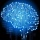Home Communities
IT Knowledge
Inspiration
Languages
EN

# MySQL - get row position with SELECT query

4 points
Created by:Root-ssh
170660

Quick solution:

``````SET @row_number := 0;

SELECT
(@row_number := @row_number + 1) AS `row_number`,
t.*
FROM `my_table` t;``````

## Practical example

Simple query:

``````SELECT
(@row_number := @row_number + 1) AS `row_number`,
`client_id`,
`creation_time`
FROM `clients`
JOIN (SELECT @row_number := 0) r``````

Warning: above query for some databases for the first query after the connection was established database may return `NULL` (e.g. MariaDB 10.3).

Screenshot:

## Alternative solutions

This approach creates a row number variable in `FROM` part.

``````SELECT
(@row_number := @row_number + 1) AS `row_number`,
t.`client_id`,
t.`creation_time`
FROM (SELECT @row_number := 0) r, `clients` t``````

Warning:

• immediately after connection was established, some databases may return `NULL` using the above query (e.g. MariaDB 10.3),
• `SELECT @row_number := 0` source should be placed as first to avoid syntax error for complex queries.

This approach creates a row number variable outside `SELECT` query.

``````SET @row_number := 0;
SELECT
(@row_number := @row_number + 1) AS `row_number`,
`client_id`,
`creation_time`
FROM `clients```````

### 3. Column partition

This approach uses a partition mechanism that lets to assign row numbers with a built-in function.

Using `ROW_NUMBER()` function with partition, we are able to assign unique row numbers per created partition. The partition can be created for any column. The partitioning mechanism tries to group rows that have the same values by indicated column and assign row numbers for rows in groups (each value creates a group that will have numbered rows from `1` to N). So, the trick is to create one global partition/group using an additional column that stores always the same value for each row - it will let to create one global group - in the below example, `empty` is the column name used to create one global partition/group. In the below case `empty` column contains `NULL` values always.

``````SELECT
ROW_NUMBER() over (PARTITION BY t.`empty`) AS `row_number`,  /* it is required to add additional `empty` column in `clients` table */
`client_id`,
`creation_time`
FROM `clients` t``````

## References

1. MySQL - find row number

## Alternative titles

Join to our subscribers to be up to date with content, news and offers.